# An Important Mathematical Oversight

The original intention for this website was to encourage public awareness of an historical medical crime, one that has remained a tightly-kept British state secret now for more than five decades. The matter is of enormous public interest, not least because the motivation behind the crime itself was that of advancing scientific research into areas that would come to provide the seminal knowledge behind much of the technological progress of the last half-century. My investigation into the matter inspired a parallel enquiry into some of the fundamental principles that underpin that scientific and technological impulse.

There are therefore two principle concerns of this website, and if there is acknowledged to be a substantive connection between them, that has inevitably to do with late 20th Century developments in science and information technologies, and more broadly with the idea of an burgeoning technocracy – the suggestion of a growing alliance between corporate technology and state power – one which might be judged to have atrophied the powers conventionally assigned to liberal-democratic institutions. This link therefore serves as a segue to emphasise the equal importance, to my mind, of what is going on in the X.cetera section of the site, so that that section should not appear, from the point of view of the other, as some kind of 'afterthought'.

X.cetera is concerned with a problem in mathematics and science to do with the way we think about numbers. As a subset of the category defined as integers, elements in the series of the natural numbers are generally held to represent quantities as their absolute, or 'integral', properties. It is argued that this conventional understanding of integers, which is the one widely held amongst mathematicians and scientists adopting mathematical principles, is the cause of a significant oversight with regard to changes in the relations of proportion between numbers when numerical values are transposed out of the decimal rational schema into alternative numerical radices, such as those of binary, octal, and hexadecimal, etc.

On the page: The Limits of Rationality it is argued that the relations of proportion between integers are dictated principally by their membership of the restricted group of characters (0-9) as defined by the decimal rational schema; and that corresponding ratios of proportion cannot be assumed to apply between numerically equal values when transposed into alternative numerical radices having either reduced (as in binary or octal, for instance) or extended (as in hexadecimal) member-ranges.

This is shown to be objectively the case by the results published at: Radical Affinity and Variant Proportion in Natural Numbers, which show that for a series of exponential values in decimal, where the logarithmic ratios between those values are consistently equal to 1, the corresponding series of values when transposed into any radix from binary to nonary (base-9) results in logarithmic ratios having no consistent value at all, in each case producing a graph showing a series of variegated peaks and troughs displaying proportional inconsistency.

These findings are previously unacknowledged by mathematicians and information scientists alike, but the import of the findings is that, while the discrete values of individual integers transposed into alternative radices will be ostensibly equal across those radices, the ratios of proportion between those values will not be preserved, as these ratios must be determined uniquely according to the range of available digits within any respective radix (0-9 in decimal, 0-7 in octal, for instance); one consequence of which of course is the variable relative frequency (or 'potentiality') of specific individual digits when compared across radices. This observation has serious consequences in terms of its implications for the logical consistency of data produced within digital information systems, as the logic of those systems generally relies upon the seamless correspondence, not only of 'integral' values when transcribed between decimal and the aforementioned radices, but ultimately upon the relations of proportion between those values.

Information Science tends to treat the translation and recording of conventional analogue information into digital format unproblematically. The digital encoding of written, spoken, or visual information is seen to have little effect on the representational content of the message. The process is taken to be neutral, faithful, transparent. While the assessment of quantitative and qualitative differences at the level of the observable world necessarily entails assessments of proportion, the digital encoding of those assessments ultimately involves a reduction, at the level of machine code, to the form of a series of simple binary (or 'logical') distinctions between '1' and '0' – positive and negative. The process relies upon a tacit assumption that there exists such a level of fine-grained logical simplicity as the basis of a hierarchy of logical relationships, and which transcends all systems of conventional analogue (or indeed sensory) representation (be they linguistic, visual, sonic, or whatever); and that therefore we may break down these systems of representation to this level – the digital level – and then re-assemble them, as it were, without corruption. Logic is assumed to operate consistently without limits, as a sort of 'ambient' condition of information systems.

In the X.cetera section I am concerned to point out however that the logical relationship between '1' and '0' in a binary system (which equates in quantitative terms with what we understand as their proportional relationship) is derived specifically from their membership of a uniquely defined group of digits limited to two members. It does not derive from a set of transcendent logical principles arising elsewhere and having universal applicability (a proposition that, despite its apparent simplicity, may well come as a surprise to many mathematicians and information scientists alike).

As the proportional relationships affecting quantitative expressions within binary are uniquely and restrictively determined, they cannot be assumed to apply (with proportional consistency) to translations of the same expressions into decimal (or into any other number radix, such as octal, or hexadecimal). By extension therefore, the logical relationships within a binary system of codes, being subject to the same restrictive determinations, cannot therefore be applied with logical consistency to conventional analogue representations of the observable world, as this would be to invest binary code with a transcendent logical potential that it simply cannot possess – they may be applied to such representations, and the results may appear to be internally consistent, but they will certainly not be logically consistent with the world of objects.

The issue of a failure of logical consistency is one that concerns the relationships between data objects – it does not concern the specific accuracy or internal content of data objects themselves (just as the variation in proportion across radices concerns the dynamic relations between integers, rather than their specific 'integral' numerical values). This means that, from a conventional scientific-positivist perspective, which generally relies for its raw data upon information derived from discrete acts of measurement, the problem will be difficult to recognise or detect (as the data might well appear to possess internal consistency). One will however experience the effects of the failure (while being rather mystified as to its causes) in the lack of a reliable correspondence between expectations derived from data analyses, and real-world events.

So that's some of what X.cetera is all about.. If you think you're 'ard enough!

Download my Open Letter to the British Prime Minister & Health Secretary
[pdf – 612KB]:The Limits of Rationality
(an important mathematical oversight)

[479KB]:Radical Affinity and Variant Proportion in Natural Numbers
[2.0MB]:Mind: Before & Beyond Computation
[470KB]:Dawkins' Theory of Memetics – A Biological Assault on the Cultural
[339KB]:Randomness, Non-
Randomness, & Structural
Selectivity

[273KB]:# Radical Affinity and Variant Proportion in Natural Numbers

The term 'Radical Affinity' stems from an investigation into the properties of natural numbers – their tendencies to behave, according to characteristics of their radices (or 'bases'), in ways previously unacknowledged in the analyses of quantitative systems. In the title page of this section I raised some concerns over conventional approaches to quantitative understanding, with respect to the definition of an 'integer', and to the principle of rational proportionality governing integers in the denotation of numeric value. This inquiry begins from an empirical comparison of values in exponential series across a limited range of diverse number radices (base-2 to base-9), in terms of the logarithmic ratios of sequential values in each exponential series, relative to the ratios of corresponding values in the decimal series. While the logarithmic ratios of sequential values in the decimal series are naturally consistent, and would produce graphs consisting of horizontal straight lines, in the case of each of the radical series reproduced here the distributions revealed are mostly irregular series of variegated peaks and troughs, displaying proportional inconsistency. In other pages in this section (and in the Analysis section below), this inquiry is treated more discursively; the following exercises attempt to explicate these concerns in basic empirical terms.

## Problematic

The following datasets are intended to explore comparisons between the decimal exponential series (100, [...], 1010) with its corresponding series in a range of radices from binary to nonary (base-9). In what follows I have used the term 'z' to refer to the exponential index, and the term 'b' to refer to the radical index or base. The decimal series is represented by sequential values of s=10z10. Values in each respective corresponding radix are represented by sequential values of s=(xz)b ; i.e., for x=1010 ; z=(0, [...], 10) ; b=(2, [...], 9). Generally, s is equal to (xz)b, and is employed here to represent the exponential series of any radix, whereas x retains association with the initial decimal value (e.g., 101).

In the decimal series, for z=(0, [...], 10), s=(1, [...], 10000000000).

The following tables show distributions of values corresponding to s=10z10 , in terms of s=(xz)b for each of the respective radices:

z s=10z10 s=1010z2 s=101z3
0 1 1 1
1 10 1010 101
2 100 1100100 10201
3 1000 1111101000 1101001
4 10000 10011100010000 111201101
5 100000 11000011010100000 12002011201
6 1000000 11110100001001000000 1212210202001
7 10000000 100110001001011010000000 200211001102101
8 100000000 101111101011110000100000000 20222011112012201
9 1000000000 111011100110101100101000000000 2120200200021010001
10 10000000000 1001010100000010111110010000000000 221210220202122010101

Table 1(a)

s=22z4 s=20z5 s=14z6 s=13z7 s=12z8 s=11z9
1 1 1 1 1 1
22 20 14 13 12 11
1210 400 244 202 144 121
33220 13000 4344 2626 1750 1331
2130100 310000 114144 41104 23420 14641
120122200 11200000 2050544 564355 303240 162151
3310021000 224000000 33233344 11333311 3641100 1783661
212021122000 10030000000 554200144 150666343 46113200 20731371
11331132010000 201100000000 13531202544 2322662122 575360400 228145181
323212230220000 4022000000000 243121245344 33531600616 7346545000 2520607101
21110002332100000 130440000000000 4332142412144 502544411644 112402762000 27726678111

Table 1(b)

Clearly, within the terms of each respective series, the ratio: sn/sn-1 is constant for each value of z:

sn/sn-1 = 1010 = (x)b

and in a graphical representation with z as the horizontal axis, would produce horizontal straight lines at y=1010 , and y=(x)b .

However, for the non-decimal series, if we calculate sn/sn-1 dividing the figures according to base-10 rules (i.e., treating them as if they were decimal values) instead of base-b rules, in each case the resulting series becomes inconsistent above a certain (variable) value of z.

Tables 2&3 below display the resulting distributions of values of (sn/sn-1)10 for each series s=(xz)b (the expression '(sn/sn-1)10' is used here simply to imply that the sequential radical values of (xz)b are divided as if they were decimal values):

(sn/sn-1)10
z [ s=1010z2 ] [ s=101z3 ] [ s=22z4 ] [ s=20z5 ]
0 - - - -
1 1010 101 22 20
2 1089.207920792 101 55 20
3 1010 107.930693069 27.454545455 32.5
4 9010.072000655 101 64.121011439 23.846153846
5 1098.781452499 107.930686774 56.392751514 36.129032258
6 1010.008080065 101.000589126 27.555447702 20
7 9010.720064805 165.161950272 64.054313251 44.776785714
8 1010.000000001 101.003496315 53.443411218 20.049850449
9 1097.912088781 104.846159379 28.524266590 20
10 9017.207279337 104.334590762 65.313129759 32.431626057

Table 2

(sn/sn-1)10
z [ s=14z6 ] [ s=13z7 ] [ s=12z8 ] [ s=11z9 ]
0 - - - -
1 14 13 12 11
2 17.428571429 15.538461538 12 11
3 17.803278689 13 12.152777778 11
4 26.276243094 15.652703732 13.382857143 11
5 17.964536025 13.729928961 12.947907771 11.075131480
6 16.207086510 20.081882857 12.007320934 11
7 16.676027065 13.294115285 12.664634314 11.622932272
8 24.415732638 15.415932157 12.477130193 11.004828431
9 17.967452971 14.436710488 12.768596866 11.048259227
10 17.818855798 14.987188276 15.300084870 11

Table 3

If we examine the ratio sn/sn-1 logarithmically, we can more simply employ subtraction rather than division in determining the series.

Generally, logbx is given by:   log10x/log10b.

As it is conventional to derive radical logarithms from decimal logarithms, we may do so for the values of s=(xz)b given in Table 1 above, which allows us to express the ratio sn/sn-1 in terms of:

r = (logbsn) – (logbsn-1)

The following 8 subsections display tables showing the values for logbs [i.e., logb(xz)b] and r for each radical data series (binary to nonary) given in Table 1, i.e., for the initial decimal value of x=10 (the linked pdf document: Radical Affinity etc. repeats the same exercises for the starting values of x from 2 to 9 – see pp.21-101). Graphical representations of the tabular data in terms of r against z are displayed as vertical and horizontal axes respectively in the following graphs (n.b. the vertical axes in these graphs are not at a constant scale).*

* Some may find it surprising, or erroneous, that the values of z in the horizontal axes in the following graphs are not aligned with the divisional markers, but between these points. I must confess to being a novice at the use of the 'chart' function in Microsoft Excel, and so did not override this default configuration when initially entering the data, which is the configuration most suitable when creating bar charts, for instance, rather than linear distributions of precise values. The subsequent decision not to override the default configuration for these graphs, resulting in an unconventional display, was made with regard to the fact that the precise alignment of integers with divisional markers is characteristic only of a certain limited definition of integers, as discrete points of value on a linear scale. With consideration to the scope of this investigation, and its limitation to the sphere of natural numbers – as 'wholes' (excluding fractions) – the resulting unaligned scale of values helps to accommodate certain roles of natural numbers in describing the (not strictly linear) apportionment of numeric value corresponding to familiar divisions in space and time, for instance. In identifying certain periods of time, or regions in space, we are accustomed to using whole numbers to represent entire periods or regions (the word 'zone' covers both uses). We may speak of 'Week 1' to cover any point in time in a particular 7-day duration, for instance; or use a numeric description for concentric zones in space, such as in the London Underground map, where 'Zone 2' describes any point between the lines of concentric division separating it from Zones 1 & 3 (stations occurring precisely on the line are understood to occupy both adjacent zones simultaneously). In physics, for instance, we might wish to consider a continuous sine wave in terms of its discrete single iterations, and then identify them consecutively as iterations 1, 2, & 3, etc., and so to dissociate the numeric notation from any particular point of amplitude of the sine wave (such a dissociation being impossible for any precise positional point on the horizontal axis). By allowing attention to such 'periodic' or 'zonal' features in certain ways of using integers we may perhaps help illuminate and further explain their apparent proportional inconsistencies.

x=10

## Binary

z s=1010z2 logbs r
0 1 0 -
1 1010 9.980139578 9.980139578
2 1100100 20.069203241 10.089063663
3 1111101000 30.049342819 9.980139578
4 10011100010000 43.186665738 13.137322919
5 11000011010100000 53.288354486 10.101688748
6 11110100001001000000 63.268505605 9.980151119
7 100110001001011010000000 76.405932289 13.137426684
8 101111101011110000100000000 86.386071867 9.980139578
9 111011100110101100101000000000 96.486618692 10.100546825
10 1001010100000010111110010000000000 109.625083660 13.138464968

log102 = 0.301029995664

Slideshow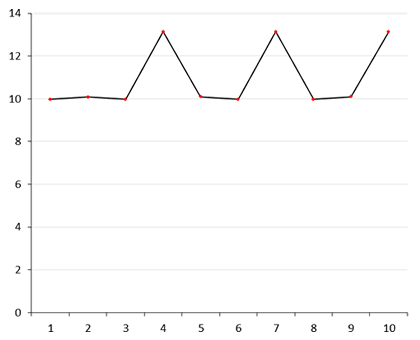r=(log2xz) – (log2xz-1), for x=10102

## Ternary

z s=101z3 logbs r
0 1 0 -
1 101 4.200863730 4.200863730
2 10201 8.401727460 4.200863730
3 1101001 12.663002651 4.261275191
4 111201101 16.863866381 4.200863730
5 12002011201 21.125141519 4.261275138
6 1212210202001 25.326010559 4.200869040
7 200211001102101 29.974535395 4.648524836
8 20222011112012201 34.175430634 4.200895239
9 2120200200021010001 38.410313290 4.234882656
10 221210220202122010101 42.640743808 4.230430518

log103 = 0.4771212547197

Slideshow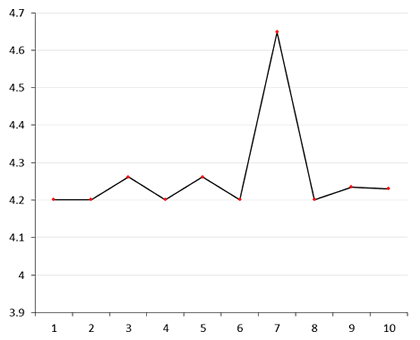r=(log3xz) – (log3xz-1), for x=1013

## Quaternary

z s=22z4 logbs r
0 1 0 -
1 22 2.229715809 2.229715809
2 1210 5.120395666 2.890679857
3 33220 7.509882226 2.389486560
4 2130100 10.511244865 3.001362639
5 120122200 13.419963781 2.908718916
6 3310021000 15.812096612 2.392132831
7 212021122000 18.812708520 3.000611908
8 11331132010000 21.682678615 2.869970095
9 323212230220000 24.099737559 2.417058944
10 21110002332100000 27.114388128 3.014650569

log104 = 0.602059991328

Slideshow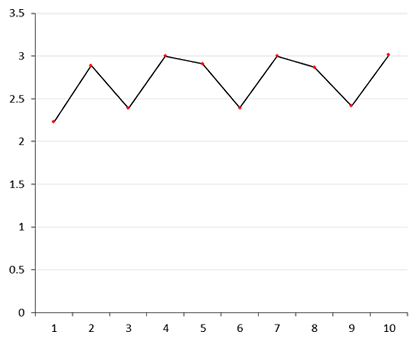r=(log4xz) – (log4xz-1), for x=224

## Quinary

z s=20z5 logbs r
0 1 0 -
1 20 1.861353116 1.861353116
2 400 3.722706232 1.861353116
3 13000 5.885722315 2.163016083
4 310000 7.856362447 1.970640132
5 11200000 10.085150978 2.228788531
6 224000000 11.946504094 1.861353116
7 10030000000 14.308626795 2.362122701
8 201100000000 16.171526676 1.862899881
9 4022000000000 18.032879792 1.861353116
10 130440000000000 20.194587325 2.161707533

log105 = 0.698970004336

Slideshowr=(log5xz) – (log5xz-1), for x=205

## Senary

z s=14z6 logbs r
0 1 0 -
1 14 1.472885940 1.472885940
2 244 3.068028002 1.595142062
3 4344 4.675042050 1.607014048
4 114144 6.499318847 1.824276797
5 2050544 8.111365354 1.612046507
6 33233344 9.665953809 1.554588455
7 554200144 11.236461588 1.570507779
8 13531202544 13.019752124 1.783290536
9 243121245344 14.631889245 1.612137121
10 4332142412144 16.239391402 1.607502157

log106 = 0.7781512503836

Slideshow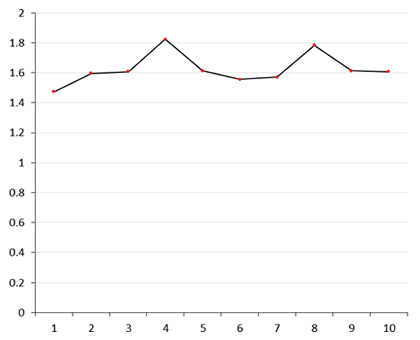r=(log6xz) – (log6xz-1), for x=146

## Septenary

z s=13z7 logbs r
0 1 0 -
1 13 1.318123223 1.318123223
2 202 2.727909971 1.409786748
3 2626 4.046033194 1.318123223
4 41104 5.459584413 1.413551219
5 564355 6.805781229 1.346196816
6 11333311 8.347382756 1.541601527
7 150666343 9.677002975 1.329620219
8 2322662122 11.082721287 1.405718312
9 33531600616 12.454713875 1.371992588
10 502544411644 13.845937269 1.391223394

log107 = 0.8450980400143

Slideshow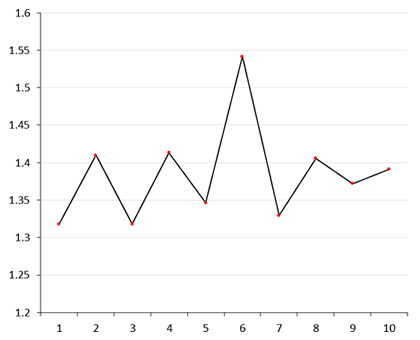r=(log7xz) – (log7xz-1), for x=137

## Octal

z s=12z8 logbs r
0 1 0 -
1 12 1.194987500 1.194987500
2 144 2.389975000 1.194987500
3 1750 3.591046402 1.201071402
4 23420 4.838484485 1.247438083
5 303240 6.070033515 1.231549030
6 3641100 7.265314311 1.195280796
7 46113200 8.486225483 1.220911172
8 575360400 9.699963563 1.213738080
9 7346545000 10.924806260 1.224842697
10 112402762000 12.236628843 1.311822583

log108 = 0.9030899869919

Slideshow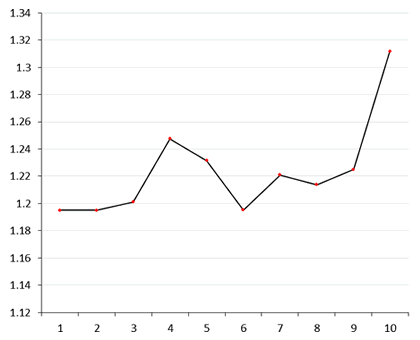r=(log8xz) – (log8xz-1), for x=128

## Nonary

z s=11z9 logbs r
0 1 0 -
1 11 1.091329169 1.091329169
2 121 2.182658339 1.091329170
3 1331 3.273987508 1.091329169
4 14641 4.365316677 1.091329169
5 162151 5.459743807 1.094427130
6 1783661 6.551072976 1.091329169
7 20731371 7.667472316 1.116399340
8 228145181 8.759001215 1.091528899
9 2520607101 9.852322719 1.093321504
10 27726678111 10.943651889 1.091329170

log109 = 0.9542425094393

Slideshow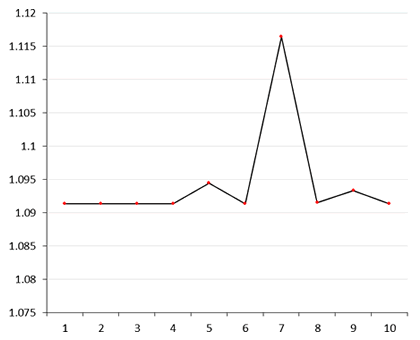r=(log9xz) – (log9xz-1), for x=119

## Proportional Graphs

The first graph below shows the distributions represented individually above, in a single graph with a proportional vertical axis for the full range b=(2, [...], 9). The three graphs in the subsequent section show the relationships between the distributions for the range b=(3, [...], 9), with expanded vertical scales (r):

Slideshowr=(logbxz) – (logbxz-1), for x=(1010)b

### Graphs to show relations of close sequential groups

Slideshow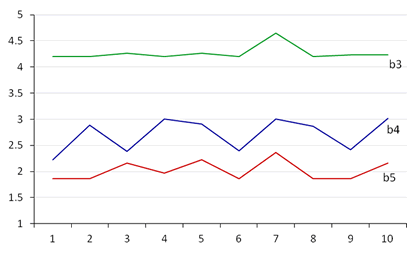Comparison of ternary, quaternary, & quinary series.

Slideshow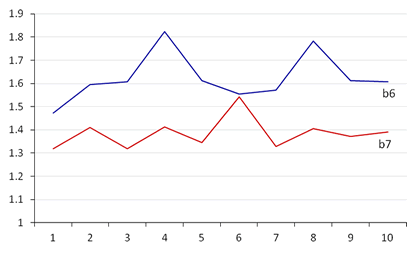Comparison of senary & septenary series.

SlideshowComparison of octal & nonary series.

## Variation Factors

To measure the degrees of variation in proportion exhibited in the values of r in each radical series, I have taken as a baseline the value of ru given for z=1, then calculated the increase factor for rv at z=7 (as this appears as a frequent point of high elevation): (rv/ru); and, if rmax > rv, the increase factor for rmax at z=n: (rmax/ru):

b ru rv rmax rv/ru (z=7) rmax/ru (z=n)
2 9.980139578 13.137426684 13.138464968 1.316   1.316 (z=10)
3 4.200863730 4.648524836 1.107 -
4 2.229715809 3.000611908 3.014650569 1.346   1.352 (z=10)
5 1.861353116 2.362122701 1.269
6 1.472885940 1.570507779 1.824276797 1.066 1.239 (z=4)
7 1.318123223 1.329620219 1.541601527 1.009 1.170 (z=6)
8 1.194987500 1.220911172 1.311822583 1.022   1.098 (z=10)
9 1.091329169 1.116399340 1.023

r=(logbxz) – (logbxz-1), for x=(1010)b

These variation factors are represented as percentage-increase as the vertical axis in the graphs below. The horizontal axis represents the value b. It is clear that the values for rv/ru and rmax/ru are virtually identical for the radices represented by b=(2, 3, 4, 5, and 9), i.e., excepting b=7, and the two adjacent radices b=6 and b=8.

rv/ru +% (z=7)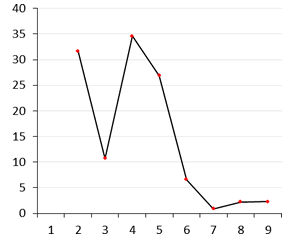rmax/ru +% (z=n)## Analysis

Much of what has been written across the other pages in this section was influenced by the findings of an earlier version of the pdf document Radical Affinity etc., which focused initially on a comparison of values in the decimal exponential sequence: 10z10, for z=(0, [...], 10), with their octal correspondents: 12z8 (see graph of the octal series). That document was then extended to similar comparisons for the series of numerical radices from binary to nonary, for values in each radix corresponding to 10 in decimal. Those exercises (presented above) reveal that, while the ratios between successive values in a progressive exponential sequence (e.g. 10z10 or 12z8) calculated by division, according to the rules of the specific radix, will be constant: (xn)b / (xn-1)b = (x)b , and would be represented graphically by a horizontal straight line at y=(x)b (i.e., with z as the horizontal axis); the treatment of the same ratios rendered through the logarithmic function (which derives all values from common logarithms – log10) results in series of ratios that are no longer constant – excepting of course those of base-10 itself. This is true for each of the numerical radices from binary to nonary, which are shown to be inconsistent with decimal, as well as being inconsistent with each other, and is evidenced by the graphs represented above, which in each case display a series of variegated peaks and troughs. Those exercises were then further extended to consider the same series of radical correspondents for the decimal values of x between 2 and 9 (see pp.21-101 of Radical Affinity etc.).

The same calculations performed for any value of x in decimal (without involving a derived radical logarithm), result in horizontal straight lines at y=Logx. In the exercises above there are no instances of horizontal straight lines; however, in later exercises, for values of x other than 1010, horizontal straight lines in general result only where the decimal value of x is equal to the value b (see for example p.22 of Radical Affinity etc., where x=2, b=2). There are three exceptions to this in the result sets: occurring where x is equal to b2 or b3 (see Comments on p.31 & p.51).

The logarithmic function was developed in the 17th century by John Napier (and later by Leonard Euler) on the premise of invariant proportion between integers, and only on this basis can the derivation of radical logarithms from 'common' logarithms be assumed to be unproblematic. For example, in the example of the octal series, the deviation from the horizontal affecting the values z=3 onwards is the result of applying logarithms to the octal series; i.e., the essential proportionality between integers on which the logarithmic function is based is lost. If we represent these ratios without the use of logarithms, i.e., by dividing successive exponentials in the series 12z8 along base-8 rules, we of course end up with a horizontal straight line at y=128. It is the derivation of the octal logarithmic values from 'common' decimal logarithms that introduces these deviations. This undermines the role of the logarithmic function in expressing common ratios of proportion across diverse number radices, and suggests that the rules of proportionality between integers apply only in a restricted sense, according to the particular range, or according to the 'group characteristics', of the select digits at our disposal. This failure inherent in the logarithmic function undermines the accepted principles of rational proportionality pertaining between diverse number radices and indicates that rationality operates effectively under formally circumscribed limits, where previously no such limits had been perceived. To account for this it is necessary to consider exactly what it is that defines an 'integer', as the behaviour of the values in the octal series, as well as in other series, suggests that integers are unable to fulfil their customary role as stable indices of intrinsic value.

A comparison of the set of all eight series may help eliminate some erroneous or misleading explanations. The most striking comparison to make in the shapes of the various distributions is that between the ternary and nonary series, as both series feature significant peaks at z=7, as well as featuring comparable smaller peaks to either side of z=7:r=(log3xz) – (log3xz-1), for x=1013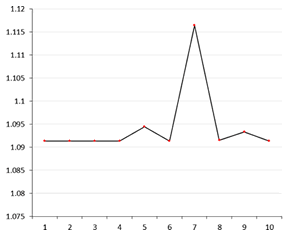r=(log9xz) – (log9xz-1), for x=119

A comparison of the two elevations at z=7 in absolute terms shows the scale of the increase in the base-9 series is only ≈5.6% of that in the base-3. However, in relative terms (i.e., taking into account the difference in the baseline value at z=1) the difference is between a ≈10.7% increase in the ternary series, and a ≈2.3% in the nonary, so that the latter increase is proportionally ≈21.6% of the size of elevation in the ternary series.

This high individual peak is not a characteristic that is repeated in the other series however, with the exception of the septenary and octal series – in the former the peak occurring at z=6, with larger adjacent peaks than those in the previous examples. The binary, quaternary, quinary, and senary series all feature more regular variations, with some signs of patterning, particularly in those of the binary and quaternary series. The octal series shows quite a unique distribution, especially in view of its elevated peak at z=10.

The statistical assessment of the variation factors with respect to the maximum (rmax) and minimum (ru) variations exhibited in each distribution, and their comparison with the variation factor at z=7 (rv), shows that in four of the eight series (b=2, 3, 5, and 9) rmax is found to occur at rv (for b=2, an equivalent maximum value recurs at z=4, 7, and 10 – the value of r at z=10 is only ≈0.008% higher than that at z=7, which I have assumed to be negligible); while in the quaternary series (b=4) the increase factor at rv is only ≈0.5% lower than its maximum, which occurs at z=10. The effect of this is that the values for rv/ru and those for rmax/ru are identical across 4/8 of the series, and almost identical across 5/8 – the exceptions being b=7 and its two adjacent radices b=6 and b=8. This similarity is displayed in the two graphs above showing percentage-increase factors for rv/ru and rmax/ru (the horizontal axis represents the value for b in these two graphs). For instance, where b=4 the value of rv is a ≈34.6% increase on the value of ru.

The identity of shape across the greater part of these two distributions (see graphs above) gives empirical proof of the qualitative uniqueness of the integer '7' (i.e., within the context of this particular series starting from the decimal x=10)1, and which is irreducible to rational or quantitative principles. A further observation is that in 3/8 of the series (b=2, b=4, and b=8) rmax occurs at z=10 (n.b., in b=2 and b=4, rmax and rv are virtually identical), so that both z=7 and z=10 appear to be 'potentiated' in comparison to other values of z. It is noticeable also that the distribution of peaks where b=2 (i.e., at z=4, z=7, and z=10) is also a characteristic found where b=4, and where b=8, although in the latter case the peaks do not exhibit the regularity of elevation found in the former two cases.

Viewing the set of distributions as a whole, it is noticeable that there is an absence of close correspondence or consistency between any two distributions (where they exhibit similarities of shape for instance, they disagree in scale). However, the statistical analysis reveals the frequency of z=7 as the point at which a half of the distributions reach maximum variation, and at which an additional one is very nearly at its maximum. The recurrent significance of z=7 and z=10 therefore seems to exclude any explanation of variation in terms of random or chaotic factors – an explanation which is also resisted by evidence of patterning in some of the distributions.

In the notations we have employed in the foregoing there are two values – z and b – which represent series of progressive whole numbers. In the conventional understanding of the meaning of an 'integer' (i.e., an entity which is self-contained, qualitatively neutral, and whose value is determined intrinsically) we should expect such series to behave proportionally. If we limit our calculations to decimal notation, there might never be an indication that integers behave in any other way (this possibly explains how these phenomena have escaped the attention of mathematicians during the 400 years since the development of Napier's method). However, in the comparisons outlined above, there are exposed proportional inconsistencies both in the sequence z=(0, [...], 10), and in the sequence b=(2, [...], 9) – the former by comparing the logarithmic differences between the sequential values in any individual radical sequence, and the latter in the absence of formal consistency between the shapes of the graphs of successive radical distributions (compare, for instance, the distributions for b=6 & b=7).

### Numbers: Transcendental Objects or Symbolic Constructs?

I have discussed elsewhere some of the theoretical problems with the concept of integers (see: Somatic Inscription & Integers & Proportion). Integers are conventionally understood to represent marks of stable intrinsic value, on the basis that any integer can be broken down, proportionally, into its constituent single units. The integrity of the unit '1' is fundamental to this understanding – it is taken as an objective unit of quantity, capable of expressing any larger quantity unambiguously by simple addition or multiplication. The problem with this understanding is that the unit '1' is not a phenomenal object with measurable properties in itself, but a symbolic construct, which 'stands-in' by a sort of tacit mental agreement as an index for value. As such it is a character that lacks a stable substantial basis, unless, that is, we assert that certain mental constructs possess transcendental objectivity. If we consider the unit '1' in the context of binary notation, for instance, we perceive that in addition to its quantitative value it is also invested with the quality of 'positivity', it being the only alternative character to the 'negative' '0'2. Can such qualitative properties be contained within the transcendental objectivity of the unit '1', considering that they do not similarly apply to '1' in decimal? In this case clearly not, as the property arises only as a condition of the restrictive binary relationship between the two digits. Hence it appears as a necessary conclusion that there are dynamic, context-specific attributes associated with particular integers which are not absolute or fixed (intrinsic), but variable, and which are determined extrinsically, according to the relative frequency of individual elements within the restricted range of available characters circumscribed by the terms of the current working numerical radix.

The results that have been elaborated above in the comparisons of diverse number radices add empirical weight to this critique. Numbers, it appears, are subject to behaviours according to their relative position within a limited series of available digits; that is, according to their relative values. It makes a difference to an instance of '1', in terms of its relative frequency, or its dispositional value, whether it is 1 in base-3 or 1 in base-10, for instance, even though 13 and 110 are quantitatively identical. I have tried to show also, in the statistical assessment of sequential radical distributions above, how this instability does not arise from random or chaotic factors, but rather determines certain patterns of affinity within a given context of variation (as exemplified by the heightened potential of z=7 within the context of x=10)3. This is the case, as I understand it, because numbers, rather than behaving as stable transcendental objects, are affected by context-specific dynamic and dispositional properties, as well as by rational principles. In fact, rational principles – the ability of numerical values to express conformable ratios – do not operate as universal principles consistently governing all numerical notations, but depend locally on the terms of the particular numerical radix we happen to be employing. The rational principles governing a decimal system are incompatible with those governing an octal or a binary one, as is clearly shown in the distributions exhibited in the foregoing exercises.

Clearly, issues of relative frequency must be determined extrinsically – from the relations between integers as notional quantities, or between the individual members of relational groups of digits (as quantities) in series. From the conventional rational (and, it has to be said, deeply metaphysical) viewpoint of integers as self-contained transcendental objects whose values are determined intrinsically, the constitutive effect of numerical behaviour upon value, of quality upon quantity, will forever be shrouded in mystery.

These criticisms are not intended to be exhaustive, but are an initial response to the problems arising from the failure of the principle of rational proportionality, as evinced by the exercises in the foregoing sections, as well as in the extended exercises in the pdf document: Radical Affinity etc. I do not suggest here that this analysis provides a full or adequate explanation of the instances of variable proportionality revealed in these exercises. Attempts at analytical explanation tend to be predisposed towards rational solutions; and after all it was necessary to employ the distinctly rational method of logarithmic comparison in order to expose the failures in rational proportionality as the principle underpinning that method.

Having got thus far, and in the light of these results, it may be that we must accept that rationality operates only within well-defined limits – that it is ultimately undermined as an overarching principle in the analysis of quantitative systems. It begins to appear as a contingent, relational property, which fluctuates according the terms of a signifying regime (i.e., according to its numeric or indexical syntax), rather than as a necessary precondition with universal, or absolute, applicability. This suggests that a fully rational explanation of these results may ultimately be unjustifiable, or inappropriate.

Further historical and epistemological enquiry should help towards an understanding of how the selective inheritance of certain notions from Classical mathematics lent support to the overvaluation of the principle of rational proportionality, by hypostatising that which should correctly be understood as a fundamentally conceptual category (number), into something idealised as a concrete entity (integer), asserting the latter as proportionally invariant, or qualitatively indifferent. A major impulse, from the 16th Century onwards, towards the mechanical understanding of the laws of Nature, required the establishment of systems of absolute measurement and quantification; which in turn enforced the rational dichotomy between quantitative and qualitative forms of knowledge. While this may have been historically and theoretically necessary to the development of modern empirical science in its infancy, from our own developed information-based technological perspective, which mistakenly assumes a seamless correspondence between diverse numerical radices (binary, octal, decimal, hexadecimal, base-64, etc.), the continued disregard for their rational non-conformability becomes an issue of urgent critical importance.

November 2011
(revised 1 October 2023)

### Footnotes:

1. The same qualitative characteristics do not apply to instances of z=7 in the series taking the decimal values of x from 2 to 9 as their starting point, as shown in the analysis of variations across these further series in pp.102-112 of the linked pdf document: Radical Affinity etc. (see also Note 3 below). [back]
2. This characteristic is of course what enables digital computer systems to employ binary code principally to convey a series of processing instructions, rather than merely as an index of quantity. [back]
3. As a further example, see the distributions of variation factors given for the series where x=9, on p.110 of Radical Affinity etc.. In this context there is displayed a heightened frequency of elevated values of r where z=6, occurring in 4/8 of the series (i.e., for b= 2, 4, 5, & 8). [back]